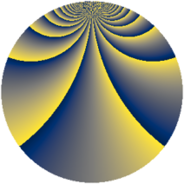# Properties

 Label 2736.2.uLevel $2736$ Weight $2$ Character orbit 2736.u Rep. character $\chi_{2736}(341,\cdot)$ Character field $\Q(\zeta_{4})$ Dimension $320$ Sturm bound $960$

# Related objects

## Defining parameters

 Level: $$N$$ $$=$$ $$2736 = 2^{4} \cdot 3^{2} \cdot 19$$ Weight: $$k$$ $$=$$ $$2$$ Character orbit: $$[\chi]$$ $$=$$ 2736.u (of order $$4$$ and degree $$2$$) Character conductor: $$\operatorname{cond}(\chi)$$ $$=$$ $$912$$ Character field: $$\Q(i)$$ Sturm bound: $$960$$

## Dimensions

The following table gives the dimensions of various subspaces of $$M_{2}(2736, [\chi])$$.

Total New Old
Modular forms 976 320 656
Cusp forms 944 320 624
Eisenstein series 32 0 32

## Trace form

 $$320q + O(q^{10})$$ $$320q - 16q^{16} + 16q^{19} - 320q^{49} + 64q^{61} - 96q^{64} - 48q^{76} + 80q^{82} + 64q^{85} + O(q^{100})$$

## Decomposition of $$S_{2}^{\mathrm{new}}(2736, [\chi])$$ into newform subspaces

The newforms in this space have not yet been added to the LMFDB.

## Decomposition of $$S_{2}^{\mathrm{old}}(2736, [\chi])$$ into lower level spaces

$$S_{2}^{\mathrm{old}}(2736, [\chi]) \cong$$ $$S_{2}^{\mathrm{new}}(912, [\chi])$$$$^{\oplus 2}$$